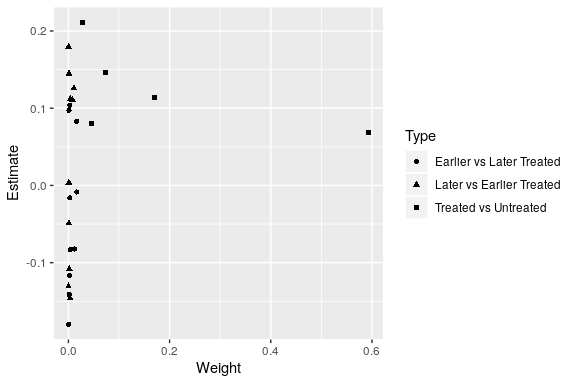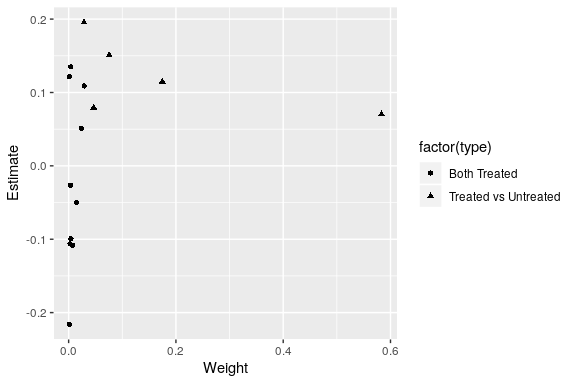# bacon

## Description

bacon() is a function that decomposes two-way fixed effects models into all 2x2 estimates and their weights following Goodman-Bacon (2019). It can perform the decomposition with and without time-varying covariates. Below are a few great references you can use to familiarize yourself with the Goodman-Bacon decomposition before using this function.

## Example: Castle Doctrine

The following example comes from Cheng and Hoekstra (2013, JHR). The authors estimate the effect of “castle doctrines,” state laws that make it easier to use lethal force in self defense. The data set castle contains state/year level information from 2000-2010 on crime rates and whether or not the state had a castle doctrine in effect. Here we replicate the analysis on homicide rates. The key variables are :

• state
• year
• l_homicide log of the homicide rate
• post indicator for whether a castle doctrine law was in effect

First, we perform the decomposition without time-varying controls, and make sure that the weighted average of the decomposition equals the two-way fixed effects estimate.

library(bacondecomp)

df_bacon <- bacon(l_homicide ~ post,
data = bacondecomp::castle,
id_var = "state",
time_var = "year")
#>                       type  weight  avg_est
#> 1 Earlier vs Later Treated 0.05976 -0.00554
#> 2 Later vs Earlier Treated 0.03190  0.07032
#> 3     Treated vs Untreated 0.90834  0.08796
coef_bacon <- sum(df_bacon$estimate * df_bacon$weight)
print(paste("Weighted sum of decomposition =", round(coef_bacon, 4)))
#>  "Weighted sum of decomposition = 0.0818"

fit_tw <- lm(l_homicide ~ post + factor(state) + factor(year),
data = bacondecomp::castle)
print(paste("Two-way FE estimate =", round(fit_tw$coefficients, 4))) #>  "Two-way FE estimate = 0.0818" Now, we plot each 2x2 estimate and its weight to see what is driving the result. library(ggplot2) ggplot(df_bacon) + aes(x = weight, y = estimate, shape = factor(type)) + labs(x = "Weight", y = "Estimate", shape = "Type") + geom_point()In this example, one estimate has almost 60 percent of the weight: states treated in 2006 vs states that are never treated. We can also perform the decomposition with time varying controls. In this example we add the log of state/year level population (l_pop) and income (l_income). A couple things to note: • When including controls there is an additional source of identifying variation that comes from within treatment timing group. It is noted as $$\hat{\beta^p_w}$$. $$\Omega$$ is the weight given to this estimate in the two-way fixed effects estimate. • The 2x2s are no longer decomposed into “early vs late” and “late vs early” estimates. For each treatment group dyad, there is only one estimate, $$\hat{\beta}^d_{b, k, l}$$ which gets weight $$s_{k, l}$$. The two way fixed effects estimate, $$\hat{\beta}^{DD|X}$$, then decomposes to: $$\hat{\beta}^{DD|X} = \Omega \hat{\beta^p_w} + (1 - \Omega) \sum_{k} \sum_{l > k} s_{k, l} \hat{\beta}^d_{b, k, l}$$ ret_bacon <- bacon(l_homicide ~ post + l_pop + l_income, data = bacondecomp::castle, id_var = "state", time_var = "year") #> type weight avg_est #> 1 Both Treated 0.09121 0.02724 #> 2 Treated vs Untreated 0.90879 0.09002 beta_hat_w <- ret_bacon$beta_hat_w
beta_hat_b <- weighted.mean(ret_bacon$two_by_twos$estimate,
ret_bacon$two_by_twos$weight)
Omega <- ret_bacon$Omega bacon_coef_cont <- Omega*beta_hat_w + (1 - Omega)*beta_hat_b print(paste("Weighted sum of decomposition =", round(bacon_coef_cont, 4))) #>  "Weighted sum of decomposition = 0.0906" two_way_fe_cont <- lm(l_homicide ~ post + l_pop + l_income + factor(state) + factor(year), data = bacondecomp::castle) two_way_fe_coef_cont <- two_way_fe_cont$coefficients["post"]
print(paste("Two way FE estimate =", round(two_way_fe_coef_cont, 4)))
#>  "Two way FE estimate = 0.0906"

And again we can plot the decomposed between estimates, $$\hat{\beta}^d_{b, k, l}$$ and their weights, $$s_{k, l}$$.

ggplot(ret_bacon\$two_by_twos) +
aes(x = weight, y = estimate, shape = factor(type)) +
labs(x = "Weight", y = "Estimate") +
geom_point()